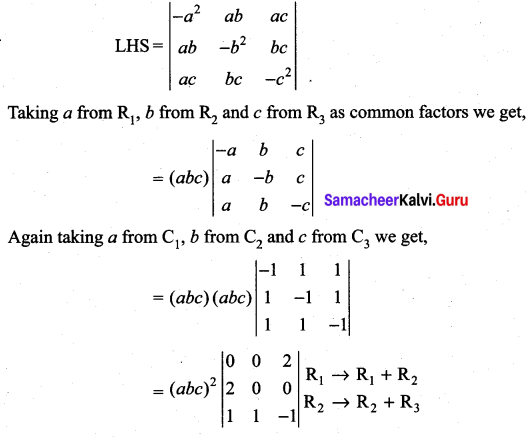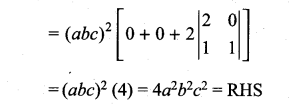## Tamilnadu Samacheer Kalvi 11th Maths Solutions Chapter 7 Matrices and Determinants Ex 7.2

11th Maths Exercise 7.2 Question 1.
Without expanding the determinant, prove that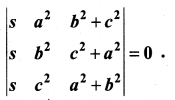Solution: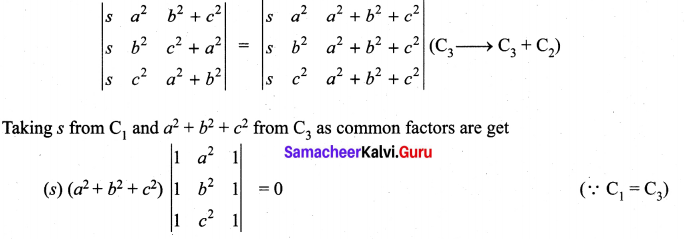11th Maths Exercise 7.2 Answers Question 2.
Show that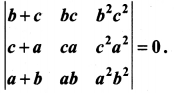Solution: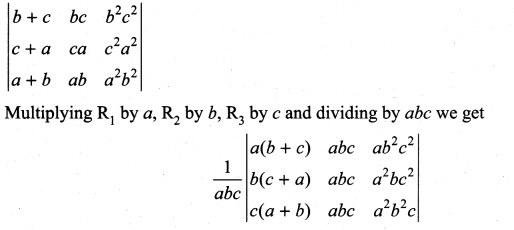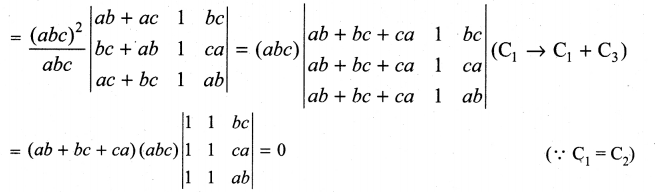Matrices And Determinants Class 11 Solutions Pdf Question 3.
Prove that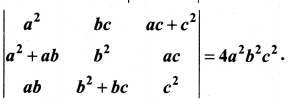Solution:
LHS
Taking a from C1, b from C2 and c from C3 we get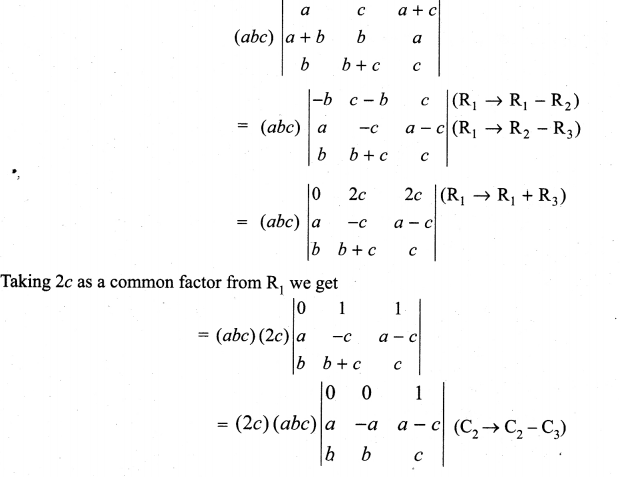Expanding along R1 we get
(2c) (abc) (1) [ab + ab] = abc (2c) (2ab)
1 = (abc) (4abc) = 4a2b2c2
= RHS

Matrices And Determinants Class 11 State Board Solutions Question 4.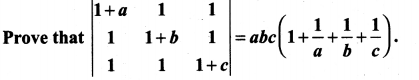Solution: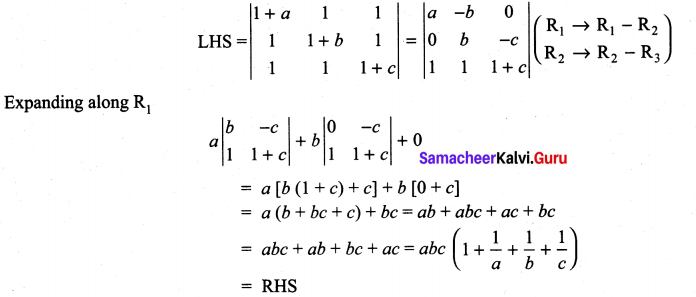11th Maths Matrices And Determinants Solutions Question 5.
Prove that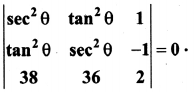Solution: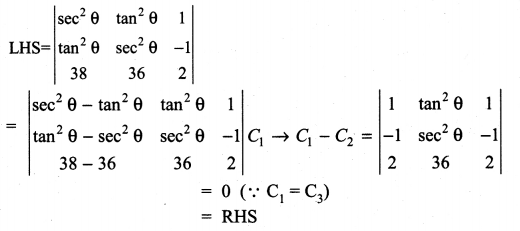11th Maths Exercise 7.2 In Tamil Question 6.
Show that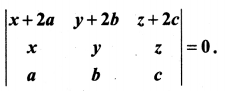Solution: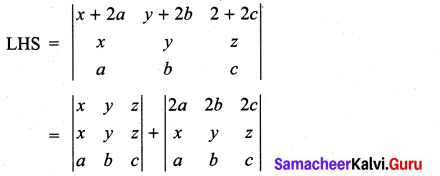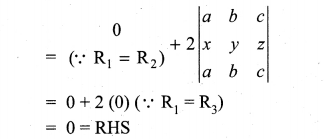11th Maths Determinants Solutions Question 7.
Write the general form of a 3 × 3 skew-symmetric matrix and prove that its determinant is 0.
Solution: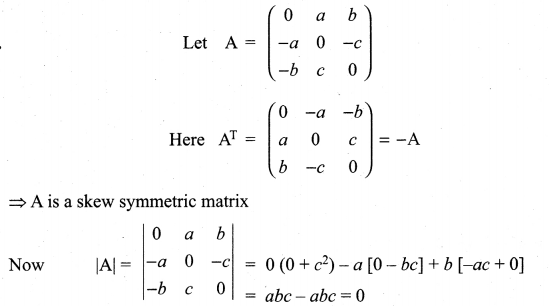11th Maths Exercise 7.2 Samacheer Kalvi Question 8.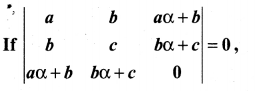Solution: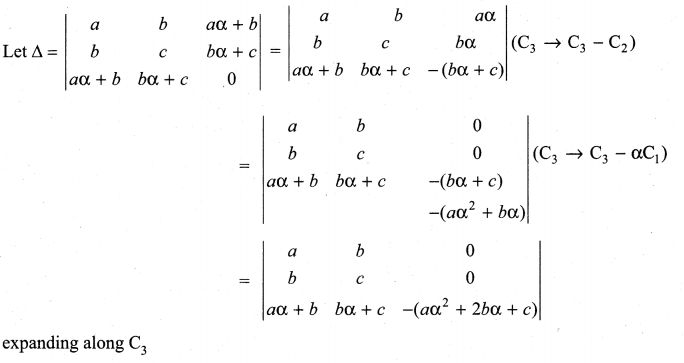we get – (aα2 + 2bα + c) [ac – b2]
So Δ = 0 ⇒ (aα2 + 2bα + c) (ac -b2) = – 0 = 0
⇒ aα2 + 2bα + c = 0 or ac – b2 = 0
(i.e.) a is a root of ax2 + 2bx + c = 0
or ac = b2
⇒ a, b, c are in G.P.

11th Maths Matrix Solutions Question 9.
Prove that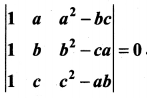Solution: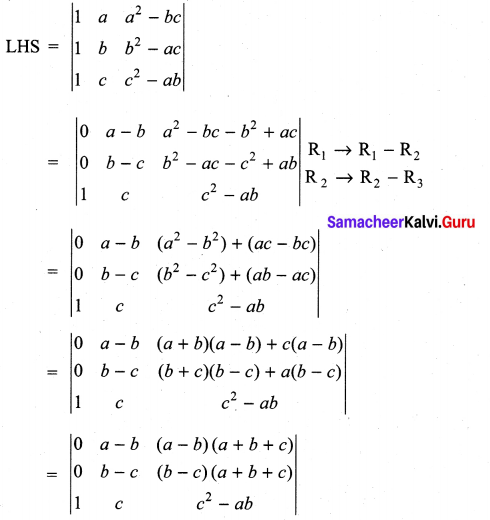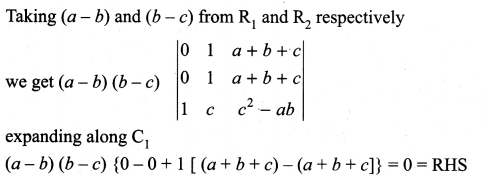Exercise 7.2 Class 11 Maths Solutions State Board Question 10.
If a, b, c are pth, qth and rth terms of an A.P., find the value of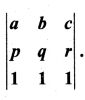Solution:
We are given a = tp,b = tq and c = tr
Let a be the first term and d be the common difference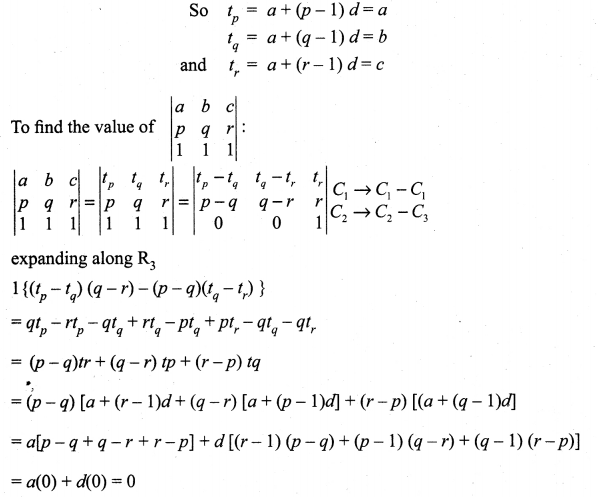11th Maths Matrix And Determinants Question 11.
Show that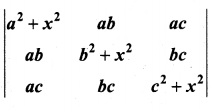is divisible by x4
Solution: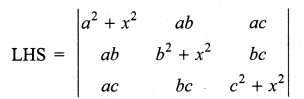Multiplying R1 by a, R2 by b and R3 by c and
taking out a from C1 b from C2 and c from C3 we get
=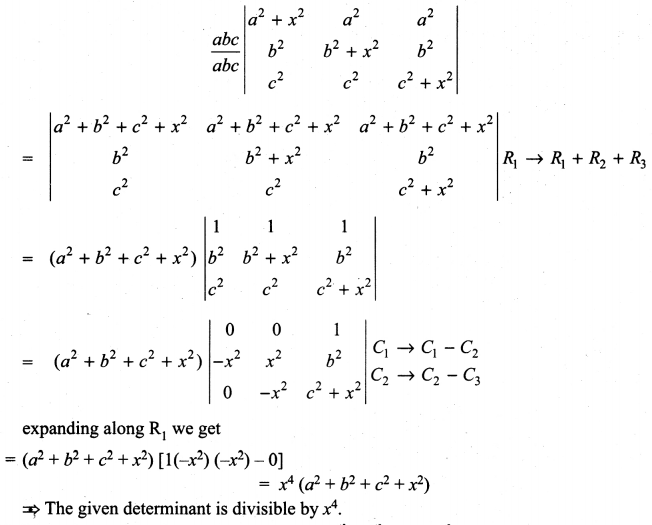=

11th Maths Ex 7.2 Question 12.
If a, b, c are all positive, and are pth, qth and rth terms of a G.P., show that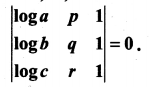Solution: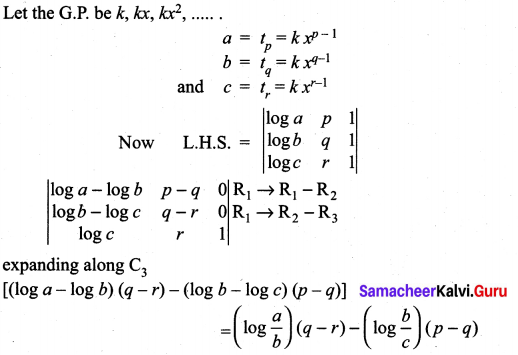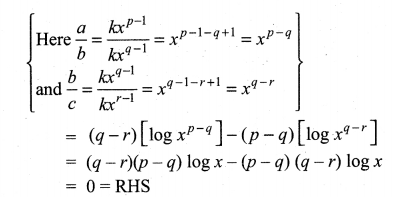Exercise 7.2 Class 11 Maths Solutions Question 13.
Find the value of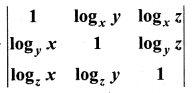if x, y, z ≠ 1.
Solution:
Expanding the determinant along R1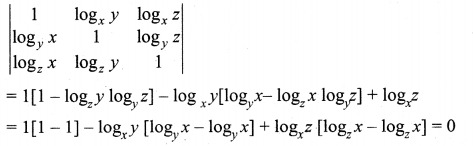11th Std Maths Determinants Solutions Question 14.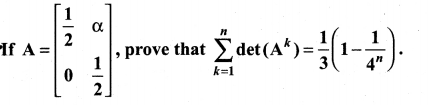Solution: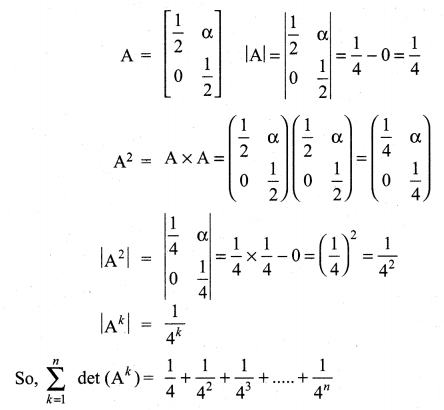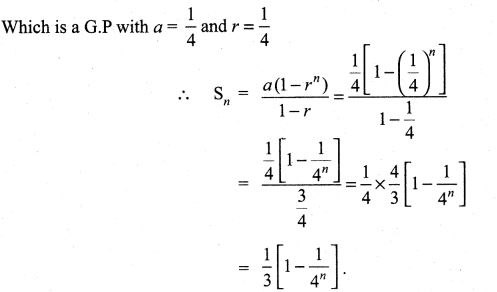11th Maths Book Volume 2 Chapter 7 Question 15.
Without expanding, evaluate the following determinants: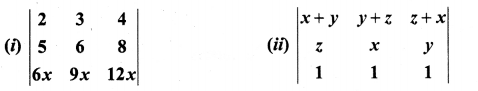Solution: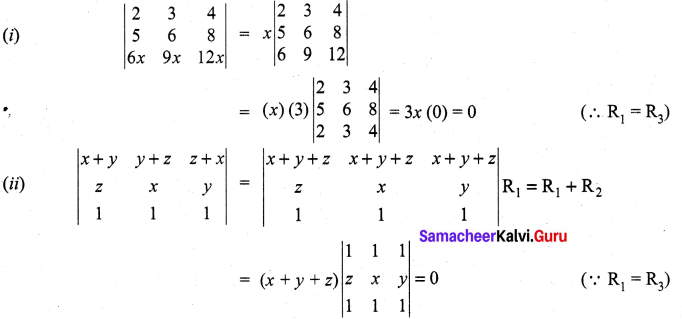10th Maths Exercise 7.2 Samacheer Kalvi Question 16.
If A is a square matrix and |A| = 2, find the value of |AAT|.
Solution:
|A| = 2 (Given) |AT| = 2
Now |AAT| = |A| |AT| = 2 × 2 = 4.

12th Maths Exercise 7.2 Samacheer Kalvi Question 17.
If A and B are square matrices of order 3 such that |A| = -1 and |B| = 3, find the value of |3AB|.
Solution:
Given |A| = -1 : |B| = 3
Given A and B are square matrices of order 3.
∴ |kAB| = k3 |AB|
Here k = 3 ∴ |3AB| = 33 |AB|
= 27 |AB|
= 27 (-1) (3)
= -81

Class 11 Maths Exercise 7.2 Solutions Question 18.
If λ = -2, determine the value of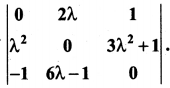Solution:
Given λ = -2
∴ 2λ = -4; λ2 = (-2)2; 3λ2 + 1 = 3 (4) + 1 = 13
6λ – 1 = 6(-2) – 1 = -13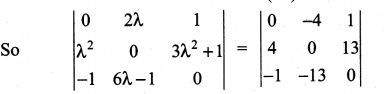expanding along R1
0(0) + 4 (0 + 13) + 1 (-52 + 0) = 52 – 52 = 0
Aliter: The determinant value of a skew-symmetric matrix is zero

Matrices And Determinants Class 11 Solutions Question 19.
Determine the roots of the equation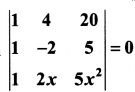Solution: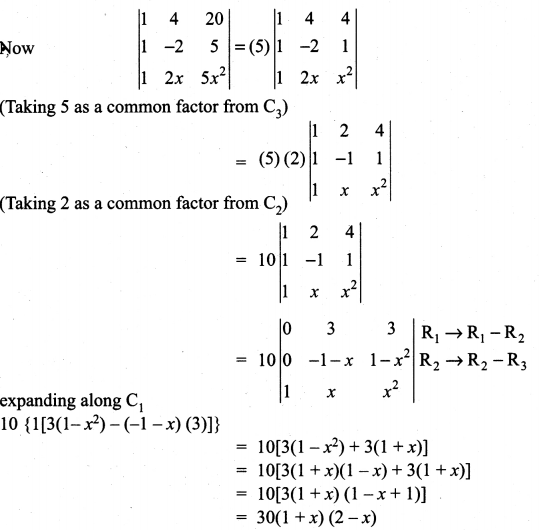Given the determinant value is 0
⇒ 30(1 + x) (2 – x) = 0
⇒ 1 + x = 0 or 2 – x = 0
⇒ x = -1 or x = 2
So, x = -1 or 2.

Matrices And Determinants Class 11 Exercise Question 20.
Verify that det (AB) = (det A) (det B) for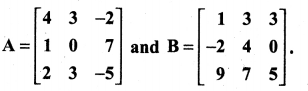Solution: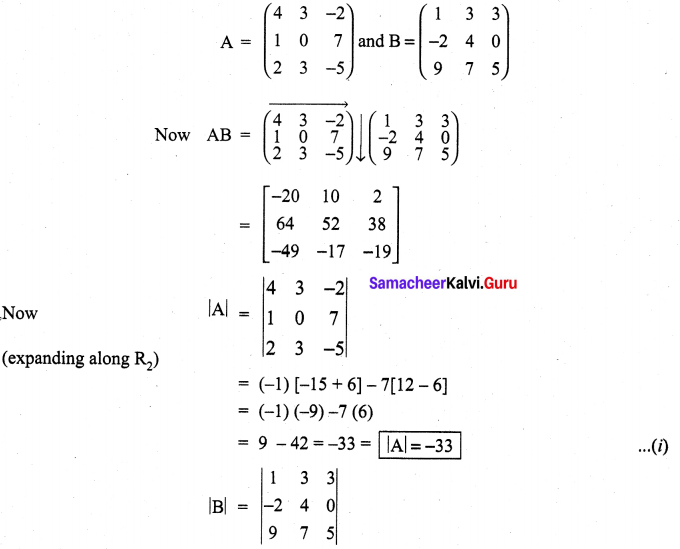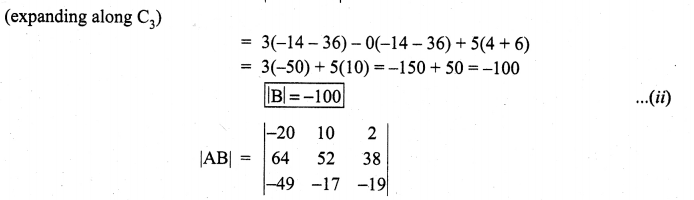{(-20)(52) (-19) + (10)(38)(—49) + (2)(64)(-17)} – {(-49)(52) (2) + (-17)(38)(-20) + (-19)(64)(10)}
= (19760 – 18620 – 2176) – (-5096 + 12920 – 12160)
= (19760 + 5096 + 12160) – (18620 + 2176 + 12920)
= 37016 – 33716 = 3300 ….(3)
Now (1) × (2) = (3)
(i.e.,) (-33) (-100) = 3300
⇒ det (AB) = (det A), (det B)

Determinants Class 11 State Board Solutions Question 21.
Using cofactors of elements of second row, evaluate |A|, where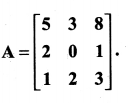Solution: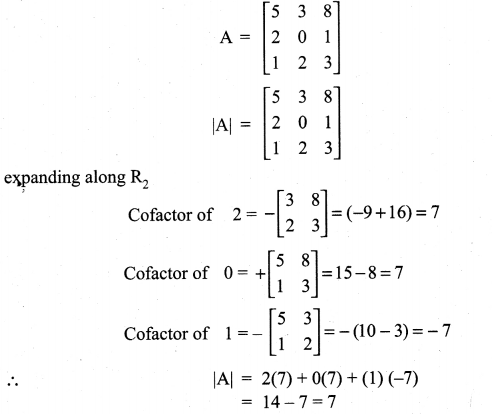### Samacheer Kalvi 11th Maths Solutions Chapter 7 Matrices and Determinants Ex 7.2 Additional Problems

Exercise 7.2 Class 10 Samacheer Kalvi Question 1.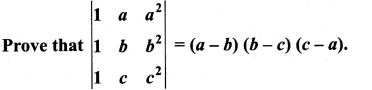Solution: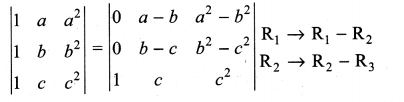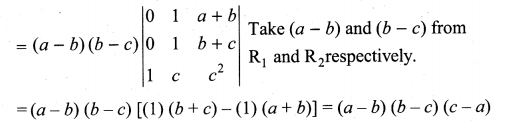11th Maths Matrices And Determinants Pdf Question 2.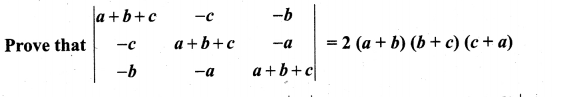Solution: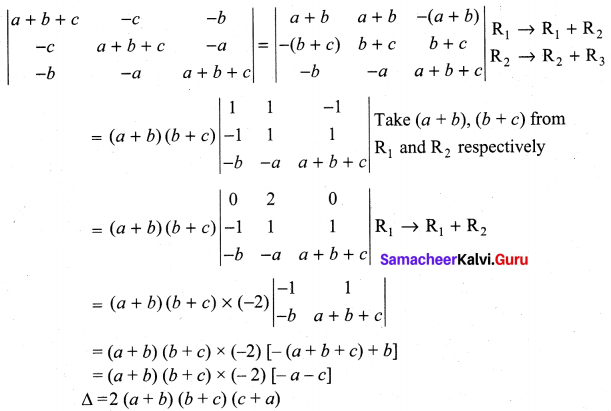Question 3.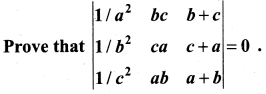Solution: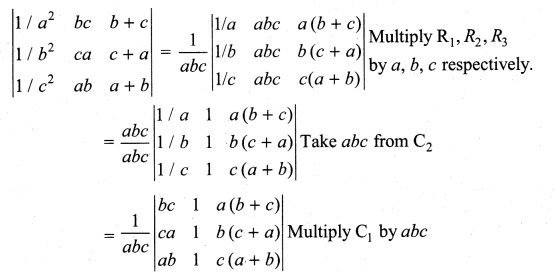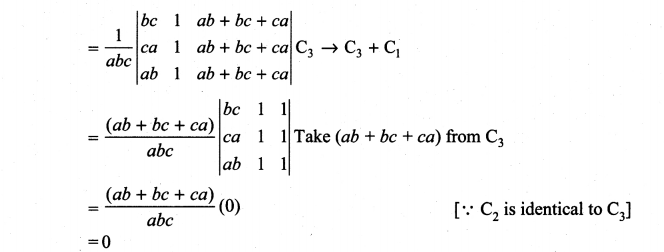Question 4.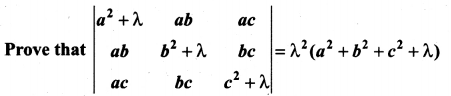Solution: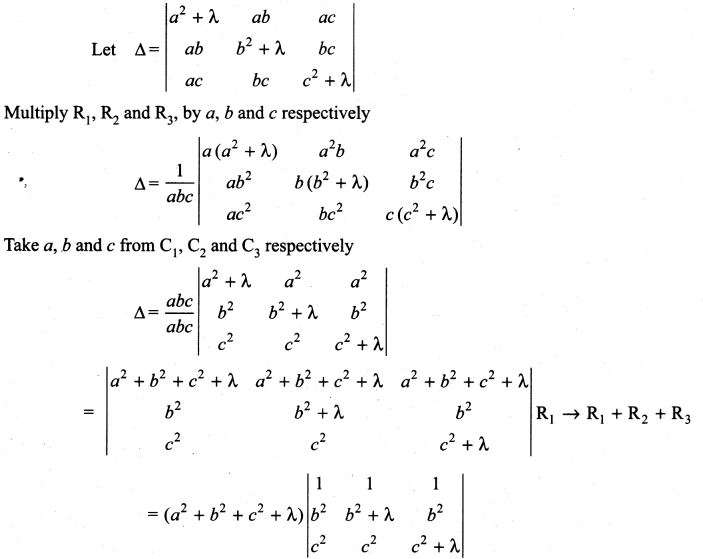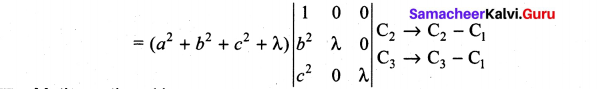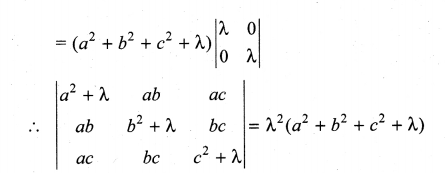Question 5.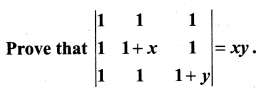Solution: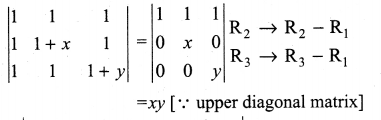Question 6.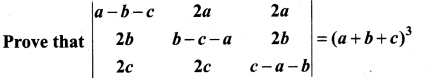Solution: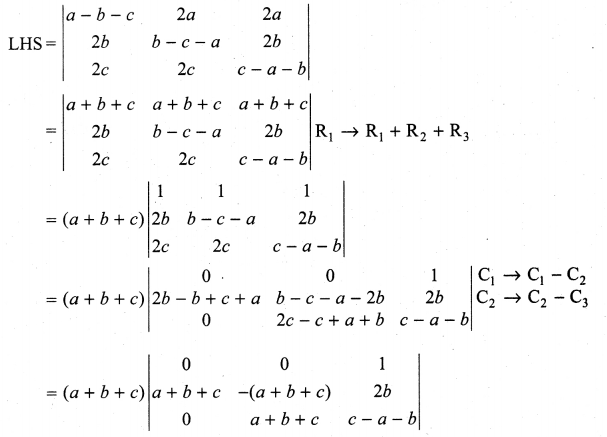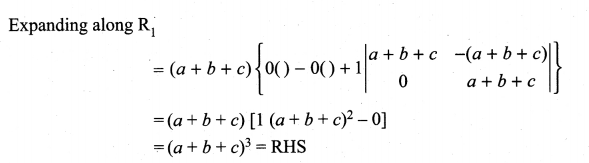Question 7.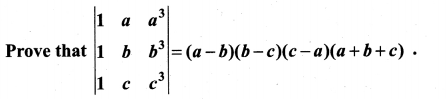Solution: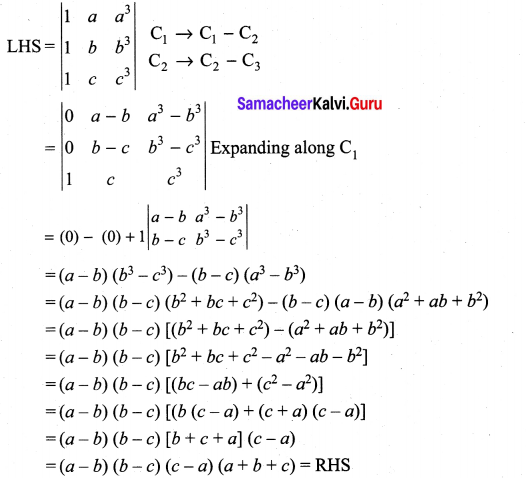Question 8.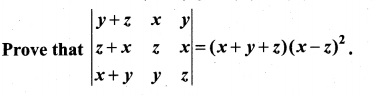Solution: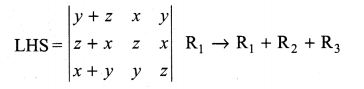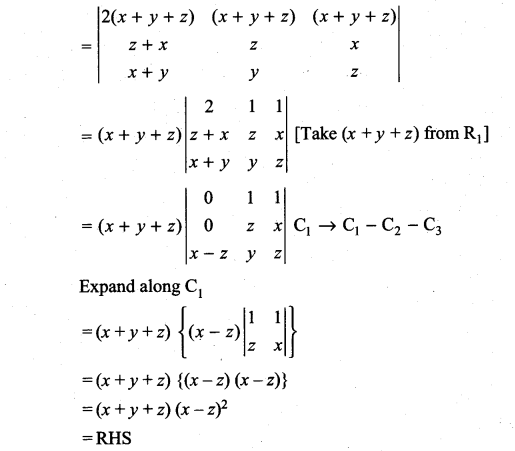Question 9.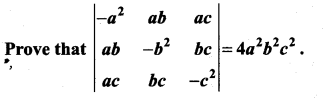Solution: